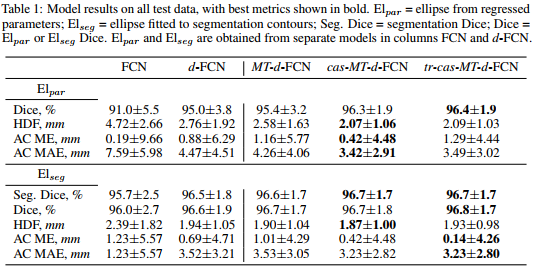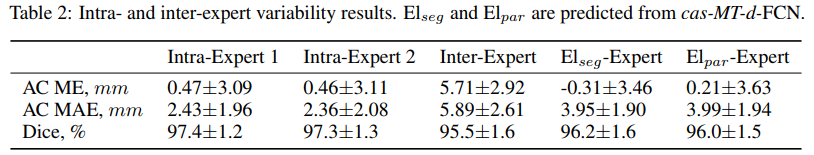# Description

In this paper, the authors propose a method to measure biometrics from fetal ultrasound (US), in particular the abdominal circumference (AC) extracted from an ellipse. To do so, they propose a simple CNN architecture that resemble an FCNN but at the same time regresses the parameters of the ellipse. C.f. Fig.1 for more details.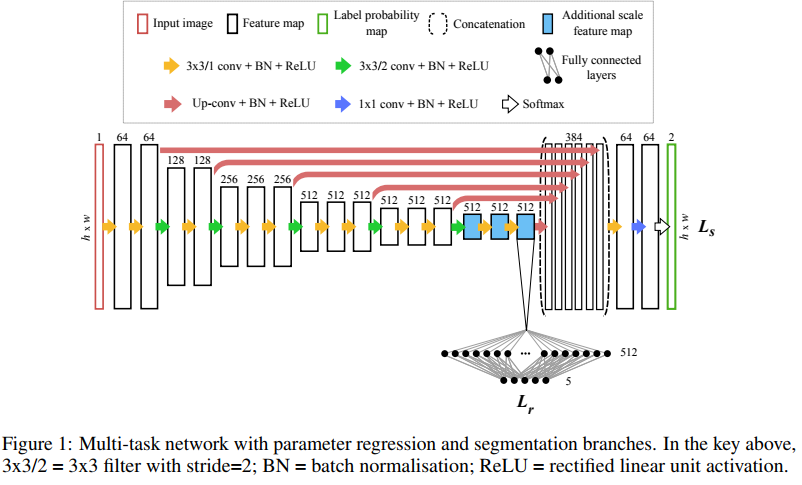# Implementation details

Since their network aims to segment the image AND recover the parameters of the ellipse, their method minimizes the following multi-task loss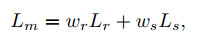where $$L_s$$ is the usual crossentropy: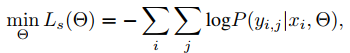and $$L_r$$ is an L2 loss of the ellipse parameters: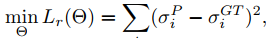where $$\sigma$$ is a 5-D vector contianing the ellipse parameters $$(a,b,c_x,c_y,\phi)$$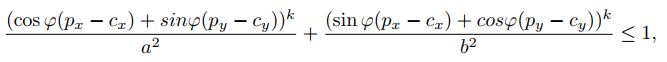In order to further improve results, they implemented a cascaded network. Instead of implementing a usual cascade (c.f. Fig.2(a)), they concatenate the output of the first network to a rotated version of the input image (c.f. Fig.2(b)).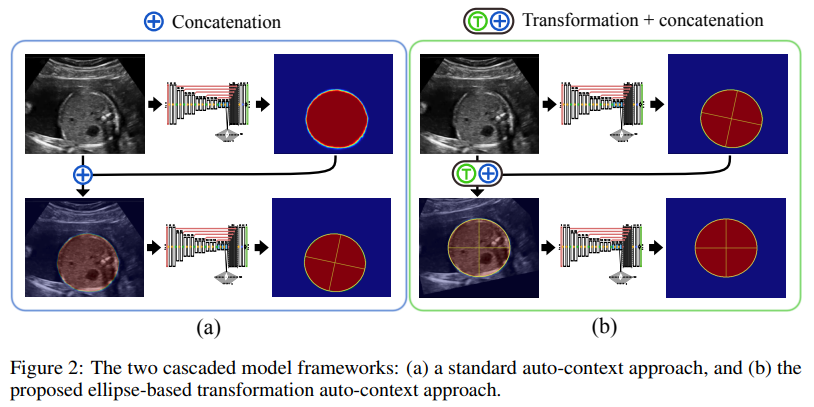# Results

Their method beats results from FCN and cascaded FCN while being within the inter-obs variation.